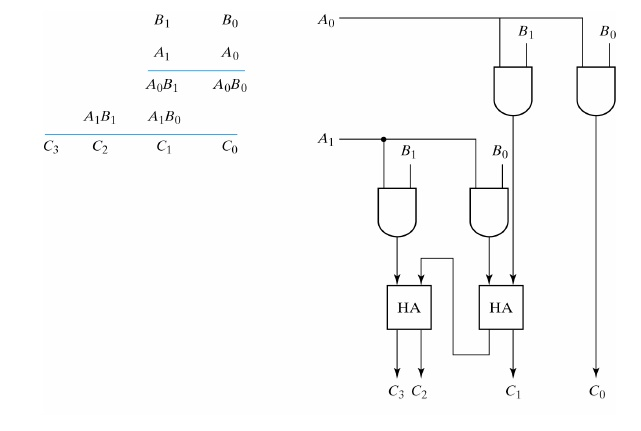Home | | Digital Electronics | Binary Multiplier

# Binary Multiplier

Multiplication of binary numbers is performed in the same way as in decimal numbers

BINARY MULTIPLIER:

Multiplication of binary numbers is performed in the same way as in decimal numbers – partial product: the multiplicand is multiplied by each bit of the multiplier starting from the least significant bitMultiplication of two bits = A * B (AND)

0 * 0 = 0 0 * 1 = 0 1 * 0 = 0 1 * 1 = 1

## 2-BIT BY 2-BIT BINARY MULTIPLIER:## 4-BIT BY 3-BIT BINARY MULTIPLIER:Study Material, Lecturing Notes, Assignment, Reference, Wiki description explanation, brief detail
Digital Electronics : Combinational Circuits : Binary Multiplier |